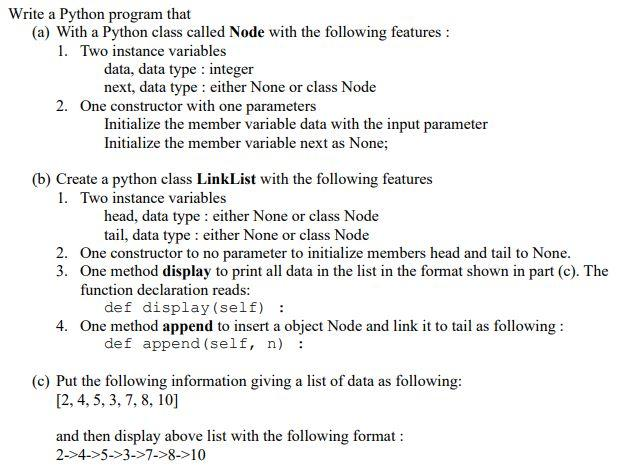# Question plz using Python to answer this question Thank ! plz using Python to answer this question Thank ! plz using Python to answer this question Thank !  plz using Python to answer this question Thank ! plz using Python to answer this question Thank !  plz using Python to answer this question Thank ! plz using Python to answer this question Thank !  plz using Python to answer this question Thank !   (a) With a Python class called Node with the following features: 1. Two instance variables data, data type : integer next, data type : either None or class Node 2. One constructor with one parameters Initialize the member variable data with the input parameter Initialize the member variable next as None; (b) Create a python class LinkList with the following features 1. Two instance variables head, data type : either None or class Node tail, data type : either None or class Node 2. One constructor to no parameter to initialize members head and tail to None. 3. One method display to print all data in the list in the format shown in part (c). The function declaration reads: def display (self) : 4. One method append to insert a object Node and link it to tail as following : def append (self, $$n$$ ) : (c) Put the following information giving a list of data as following: $$[2,4,5,3,7,8,10]$$ and then display above list with the following format :WW4OAX The Asker · Computer Science

plz using Python to answer this question Thank ! plz using Python to answer this question Thank !

plz using Python to answer this question Thank !  plz using Python to answer this question Thank !plz using Python to answer this question Thank !  plz using Python to answer this question Thank !

plz using Python to answer this question Thank !  plz using Python to answer this question Thank !

Transcribed Image Text: (a) With a Python class called Node with the following features: 1. Two instance variables data, data type : integer next, data type : either None or class Node 2. One constructor with one parameters Initialize the member variable data with the input parameter Initialize the member variable next as None; (b) Create a python class LinkList with the following features 1. Two instance variables head, data type : either None or class Node tail, data type : either None or class Node 2. One constructor to no parameter to initialize members head and tail to None. 3. One method display to print all data in the list in the format shown in part (c). The function declaration reads: def display (self) : 4. One method append to insert a object Node and link it to tail as following : def append (self, $$n$$ ) : (c) Put the following information giving a list of data as following: $$[2,4,5,3,7,8,10]$$ and then display above list with the following format :
More
Transcribed Image Text: (a) With a Python class called Node with the following features: 1. Two instance variables data, data type : integer next, data type : either None or class Node 2. One constructor with one parameters Initialize the member variable data with the input parameter Initialize the member variable next as None; (b) Create a python class LinkList with the following features 1. Two instance variables head, data type : either None or class Node tail, data type : either None or class Node 2. One constructor to no parameter to initialize members head and tail to None. 3. One method display to print all data in the list in the format shown in part (c). The function declaration reads: def display (self) : 4. One method append to insert a object Node and link it to tail as following : def append (self, $$n$$ ) : (c) Put the following information giving a list of data as following: $$[2,4,5,3,7,8,10]$$ and then display above list with the following format :